﻿ A Unified Determination of the Characteristics of Cubic Lattices

# Journal of Material Sciences & EngineeringOpen Access

All submissions of the EM system will be redirected to Online Manuscript Submission System. Authors are requested to submit articles directly to Online Manuscript Submission System of respective journal.
• Short Communication
• J Material Sci Eng 2019, Vol 8(4): 532

# A Unified Determination of the Characteristics of Cubic Lattices

Houari A1 and Alkahtani EA1,2*
1Laboratory of Theory Physics, Department of Physics, Sciences Faculty, University of Abou Bekr Belkaïd, Algeria
2Department of Physics, Khawlan Education Faculty, Sana'a University, Yemen
*Corresponding Author: Alkahtani EA, Department of Physics, Khawlan Education Faculty, Sana'a University, Algeria, Tel: 967-771960069, Email: [email protected]

Received Date: Jun 20, 2019 / Accepted Date: Jul 30, 2019 / Published Date: Aug 05, 2019

### Abstract

The purpose of this article is to develop a unified algorithmic approach for determining the characteristics of cubic lattices. For this, we will establish here the correlations between these characteristics and show that they can be expressed in terms of only two independent parameters, namely, the coordination number and the lattice constant. The main utility of the present approach consists in generating automatically the whole set of the characteristics of cubic lattices.

#### Introduction

Crystallography is a fundamental cross-disciplinary scientific field. It encompasses a wide range of scientific disciplines including condensed matter physics, solid chemistry, metallurgy and materials science. The teaching of crystallography is usually introduced early in any general textbook of the above-mentioned disciplines due to the fact that many physical properties of certain materials are directly linked to their crystalline structure. Because of the importance of crystallography in those disciplines, its teaching must be made as simple and manageable as possible. This is particularly so since, in the practice of crystallography, many physics and chemistry textbooks frequently assign exercises and problems to determine the geometrical characteristics of crystal structures separately i.e. ignoring correlations between them. This will result in a fragmentary approach involving a lot of computational redundancy. Therefore, it is pedagogically very desirable to avoid this by reducing the calculations involved in obtaining these characteristics. To contribute to this goal, we will develop in this article an algorithmic approach to determine the characteristics of cubic lattices in a unified and systematic way. The focus here is on the cubic crystal system because many solid materials including most of the metals crystallize in it.

#### Basic Crystallographic Concepts

Recall that atoms in many solid materials self-organize in crystals. Examples of these are metals, diamond, ice and graphite. In crystallography, the crystalline lattice is a periodic array of physical entities such as atoms, ions or molecules. Each crystalline lattice is characterized by a few parameters. Among these, the coordination number, usually denoted by Z, is an important geometrical characteristic giving the number of nearest neighbors of each point in the lattice. The unit cell of a crystalline lattice is the smallest structure that repeats itself by translation through the crystal. The most common types of unit cells in crystallography are the faced-centered cubic (FCC), the bodycentered cubic (BCC) and the hexagonal close-packed. The simple cubic (SC) structure is often used for didactical purpose; no material has this structure except the metal polonium. To discuss crystalline structures, it is useful to consider atoms as hard spheres i.e. sphere that just touch without overlapping, with well-defined radii. In this scheme, the shortest distance between two like atoms is twice the atomic radius. The volume occupied by a single atom in the crystal is called the atomic volume. One important characteristic of a crystalline structure is its packing fraction. It gives the proportion of space actually being used by the atoms inside the crystal. For one-component crystals i.e. crystals containing only one type of atom, the packing fraction is defined as the ratio of the total volume of atoms inside a unit cell to the volume of the latter. Mathematically, it is given by: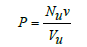(1)

where v is the atomic volume, Nu the number of atoms in the unit cell and Vu the volume of the latter. For an illustrative purpose, I will expose here the traditional procedure for calculating the packing fraction of a given lattice. For this, I will take the particular case of the FCC structure shown in Figure 1. Note that for the FCC structure, Nu=4 and the atomic radius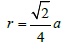with a being the length of the unit cell edge, denoted by s in Figure 1. Recall that a, called the lattice constant, is a physical characteristic of the cubic crystal structure which is experimentally determined by X-ray diffraction measurements. Replacing v and Vu by their respective expressions v=4πr3/3 and Vu=a3 in eqn. (1), the packing fraction for the FCC structure is then given by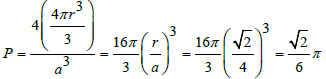For reference, the characteristics of the three cubic lattices are summarized .

Figure 1: The face-centered cubic structure has the atoms that touch along the face diagonal.

A unified approach to cubic crystallography

In examining the table referenced above, one can easily check that the number of lattice points per unit cell Nu in the three cubic lattices is simply correlated to the coordination number Z as Z ‒ 2Nu=4. Therefore, the generating function of Nu in cubic lattices is given by: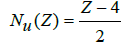(2)

By establishing eqn. (2), one aims to establish further analytical functions relating other characteristics of cubic lattices to the coordination number Z. For this, from the same table mentioned above, one first rewrites the ratio λ of the atomic radius r to the lattice constant a i.e. λ=r/a such that the parameter λ2=(4 λ)2 takes the values 4, 3 and 2 for the SC, BCC and FCC lattices respectively. Similarly, one can establish here that the coordination number Z in the three cubic lattices is correlated to the parameter λ2 as Zλ2=24 or equivalently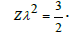The generating function of λ in cubic lattices is then given by: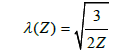(3)

Replacing v and Vu by their above expressions in eqn. (1), the packing fraction P becomes in terms of Nu and λ as: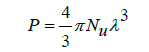(4)

By using eqns. (2) and (3), P can be expressed in terms of Z as: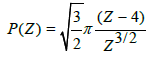(5)

One can also check here that the four characteristics Z, Nu, λ and P, in the three cubic lattices, obey the following relation:

PZ=2π Nu λ (6)

Being mathematically analogous to the well-known state equation PV=RnT characteristic of an ideal gas . This mathematical analogy will help in remembering the form of eqn. (6).

Now, from eqn. (3), one can deduce the atomic radius r in terms of Z and a as: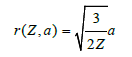(7)

A frequently calculated physical characteristic of crystalline materials is their atomic number density. It is defined as the number of atoms or formula units per unit volume of the material. Crystallographically, it is given by: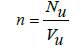(8)

Using eqn. (2) and replacing Vu by its above expression in eqn. (8), the atomic number density n in a cubic lattice can be expressed in terms of Z and a as: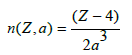(9)

Another frequently calculated physical characteristic of crystalline materials is their mass density, usually denoted by ρ, which can be defined at the atomic scale as: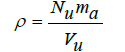(10)

where mais the mass of a single atom of the material. By definition, ma is equal to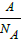where A is the atomic mass and NA Avogadro's number. Therefore, eqn. (10) becomes: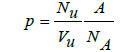(11)

Using eqn. (8), ρ will relate to n as: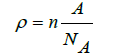(12)

Using the expression of n given by eqn. (9), the mass density ρ of a cubic lattice can be expressed in terms of Z and a as: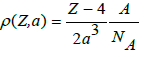(13)

Another variant of eqn. (13) written in the following form: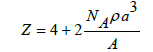(14)

will help in determining experimentally the coordination number Z to identify the type of the cubic lattice of the considered crystalline material whose ρ in eqn. (14) is now its measured mass density by any standard experimental method.

For an automatic generation of the characteristics of cubic lattices defined here, one can write a short program using one's preferred programming language. A typical Mathematical procedure for that purpose is shown in Figure 2. The output of this procedure is displayed as a table given by Figure 3.

Figure 2: A Mathematica procedure for generating the characteristics of cubic lattices.

Figure 3: Characteristics of cubic lattices generated by the Mathematica procedure shown in Figure 2.

#### Conclusion

The present approach is not intended to replace the traditional method in determining the characteristics of cubic lattices. The traditional method, which is purely geometrical, must be first performed confidently by students using their geometry knowledge, and only after that, they will be allowed to use this approach as an alternative shortcut way to solve problems in cubic crystallography. The advantage of this approach is that students need only to memorize the relevant generating functions of these characteristics and to determine correctly the coordination numbers of cubic lattices in order to get the desired results.

#### References

Citation: Houari A, Alkahtani EA (2019) A Unified Determination of the Characteristics of Cubic Lattices. J Material Sci Eng 8: 532.

Copyright: © 2019 Houari A, et al. This is an open-access article distributed under the terms of the Creative Commons Attribution License, which permits unrestricted use, distribution, and reproduction in any medium, provided the original author and source are credited.

Select your language of interest to view the total content in your interested language

##### Recommended Journals
Viewmore
###### Article Usage
• Total views: 331
• [From(publication date): 0-0 - Nov 21, 2019]
• Breakdown by view type
• HTML page views: 294Can't read the image? click here to refresh